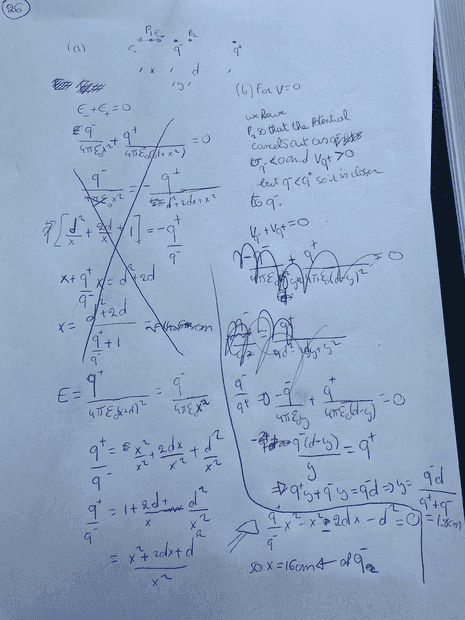# Calculating the point where potential V = 0 (due to 2 charges)

So for real world cases you need to find a function that goes to infinity as the distance from the charges gets large, and that's what I'm trying to do.

Homework Statement
Two point charges, and are placed
5.0 cm apart on the x axis. At what points along the x axis is
(a) the electric field zero and (b) the potential zero? Let
V = 0 at r = infinity.
Relevant Equations
Electric potential
Apparently, there are two solutions where the electric potential is zero which I don't understand, can I get some input on how this is possible?
I have one thing in mind (which I just thought of and might solve it), the equipotentiality i.e. when I draw a circle for V = 0 around the negative charge it will have 2 points where V = 0 one to the right and one to the left because the equipotential lines are continuous. Is that reasoining correct?I'm not able to read your photo very well. The problem statement does not say what the magnitudes of the 2 charges are.

Also, please have a look at the "LaTeX Guide" link below the Edit window. Posting math equations in LaTeX is *much* more readable that posting dim photos of your hand-written work (and is required by the PF rules). Thanks.

•nasu
Homework Statement:: Two point charges, and are placed
5.0 cm apart on the x axis. At what points along the x-axis is
(a) the electric field zero and (b) the potential zero? Let
V = 0 at r = infinity.
Relevant Equations:: Electric potential

Apparently, there are two solutions where the electric potential is zero which I don't understand, can I get some input on how this is possible?
I have one thing in mind (which I just thought of and might solve it), the equipotentiality i.e. when I draw a circle for V = 0 around the negative charge it will have 2 points where V = 0 one to the right and one to the left because the equipotential lines are continuous. Is that reasoining correct?
View attachment 301419
There must be two solutions in principle because you got a quadratic, and your argument is basically sound, but there will be degenerate cases. E.g. if the charges are equal and opposite then one solution goes to infinity.